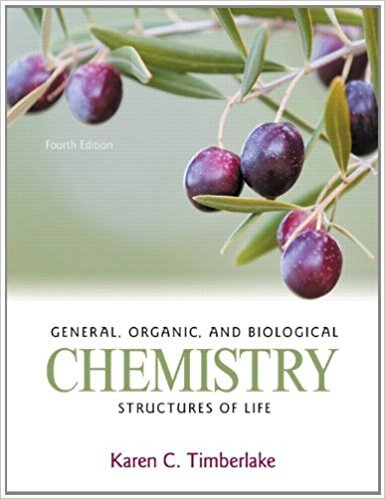# Calculate each of the following:a. moles of Al in 3.26 ×## Problem 23QP Chapter 6

General, Organic, and Biological Chemistry: Structures of Life | 4th Edition

• 2901 Step-by-step solutions solved by professors and subject experts
• Get 24/7 help from StudySoup virtual teaching assistantsGeneral, Organic, and Biological Chemistry: Structures of Life | 4th Edition

4 5 0 316 Reviews
27
3
Problem 23QP

Calculate each of the following:a. moles of Al in 3.26 × 1024 atoms of Alb. moles of C2H5OH in 8.50 × 1024 molecules of C2H5OHc. moles of Au in 2.88 × 1023 atoms of Au

Step-by-Step Solution:

Solution 23QPHere, we have to calculate the number of moles in each of the following.Step 1 of 4To calculate the number of atoms or molecules of a substance is as follows.* Convert moles to atoms or molecules.* Use Avogadro’s number writes the conversion factors.* Calculate the number of atoms.______________________________________________________________________________Step 2 of 4a.Given atoms of Al - 3.26 x 1024 atoms.Convert moles to atoms or molecules.1 mole of Al contains Avagadro’s number of Al atoms.Use Avogadro’s number writes the conversion factors. 1 mole of Al= 6.023 x 1023 atoms.So, the conversion factors are and Calculate the number of moles.3.26 x 1024 atoms = 5.42 moles of Al .Therefore, 5.42 moles of Al in 3.26 x 1024 Al atoms.________________________________________________________________________-

Step 3 of 4

Step 4 of 4

##### ISBN: 9780321750891

×
Get Full Access to General, Organic, And Biological Chemistry: Structures Of Life - 4 Edition - Chapter 6 - Problem 23qp

Get Full Access to General, Organic, And Biological Chemistry: Structures Of Life - 4 Edition - Chapter 6 - Problem 23qp

I don't want to reset my password

Need help? Contact support

Need an Account? Is not associated with an account
We're here to help